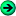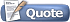Thread: Sliding weights View Single Post9th July 2005, 05:21 PM #59 Rivkin Member   Join Date: Dec 2004 Posts: 655Hi Ian, I don't think rod vs. string makes too much of a difference. 1. Equations are basically the same - they are basically the same for all oscillations. What is important is that we have oscillations around the equilibrium. Exapnding potential in Taylor's series, and taking derivative with respect to the displacement (which is going to give us force), we'll se that constant force is not there due to the equilibrium requirement (there are no forces in equilibrium, the derivative of energy is zero), force linearly proportional to the displacement is what gives us oscillations, force proportional to the displacement squared exist only in anisotropic bodies (asymmetric problem), cube will give us a nonlinear oscillator, and that is something we don't whant to deal with. So it's always restoring force linearly proportional to the displacement. 2. Now the shape determinces boundary conditions - but if something is very long in one dimension, since if it would be infinite, it would have plane waves as a solution, if it's just long it has something similar to plane waves - sin or cos (basically sum of 2 plane waves propagating in the opposite directions). Now for other dimensions - if it's a rod, it's most likely going to have a Bessel function or something like this (since it's like a drum). I think the problem is somewhere in the books on diff. equations. I think plank is more suitable than rod in case of swords, but again - we are interested in transverse oscillations along the longest dimension. Concerning additional b.c. - I meant that the tang is coupled to a human hand, so it's either unmovable, but under stress, or it's coupled to an oscillator. I still don't agree to the rest of the things... Sincerely yours, Kirill Rivkin